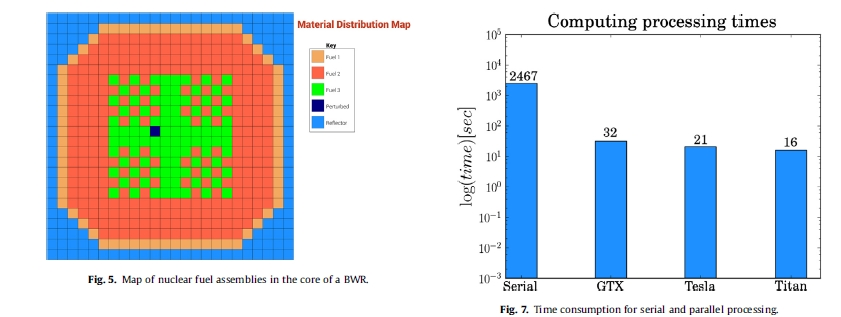# HPC implementation in the time-dependent neutron diffusion code AZKIND

#### En este artículo publicado en Annals of Nuclear Energy 99 (2017) 174 – 182 se presenta un resumen del desarrollo del código AZKIND con una implementación HPC para acelerar el cálculo de problemas numéricamente grandes.

Abstract

This article presents a summary of the development of the computer code AZKIND. This code is based on multi-group neutron diffusion theory, where the kinetics equations include delayed neutron precursors. For space discretization a Galerkin process is applied using a nodal finite elements method, and the well-known h-method is used for time discretization. A high performance computing methodology was implemented in AZKIND to solve the resulting linear algebraic system A~ 1⁄4 ~ where numerical solution of large v b algebraic systems representing full nuclear reactor cores is achieved with an acceleration technique based on the open source linear algebra PARALUTION library. This implementation allows AZKIND threading into a GPU thousands of arithmetic operations for parallel processing. The acceleration is demonstrated with the use of different nuclear fuel arrays resulting in extremely large matrices, getting a speedup ratio up to 48. Finally, the acceleration capabilities of the HPC implementation are presented in the simulation of a power transient in an actual boiling water reactor core.El trabajo se puede descargar en el siguiente link: 2017_azkind